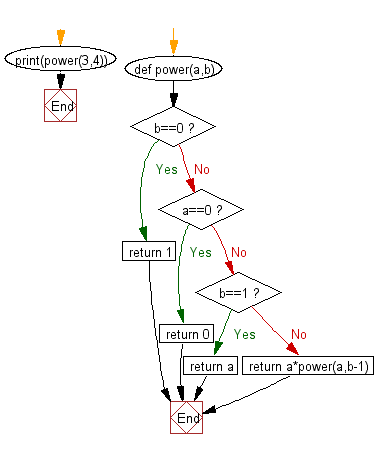﻿ Python Data Structures and Algorithms - Calculate the value of 'a' to the power 'b' - w3resource# Python Data Structures and Algorithms - Recursion: Calculate the value of 'a' to the power 'b'

## Python Recursion: Exercise-10 with Solution

Write a Python program to calculate the value of 'a' to the power 'b'.

Test Data:
(power(3,4) -> 81

Sample Solution:-

Python Code:

``````def power(a,b):
if b==0:
return 1
elif a==0:
return 0
elif b==1:
return a
else:
return a*power(a,b-1)

print(power(3,4))
```
```

Sample Output:

```81
```

Flowchart:## Visualize Python code execution:

The following tool visualize what the computer is doing step-by-step as it executes the said program:

Python Code Editor:

What is the difficulty level of this exercise?

Test your Programming skills with w3resource's quiz.

﻿

## Python: Tips of the Day

Decapitalizes the first letter of a string:

Example:

```def tips_decapitalize(s, upper_rest=False):
return s[:1].lower() + (s[1:].upper() if upper_rest else s[1:])
print(tips_decapitalize('PythonTips'))
print(tips_decapitalize('PythonTips', True))
```

Output:

```pythonTips
pYTHONTIPS
```